### Home > CAAC > Chapter 1 > Lesson 1.1.3 > Problem1-23

1-23.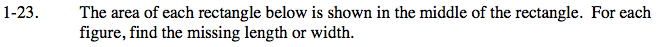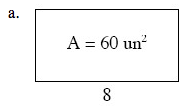The area of a rectangle is the product of the length and width.

Substitute the area and the unknown length or the width into the equation A = l · w.

Solve for the unknown variable.

$60 =\text{length} \cdot 8$

$\frac{ 60 }{ 8} = \frac{ \text{length } \cdot 8 }{8 }$

$\text{ length} = \frac{ 60 }{8 } = 7.5$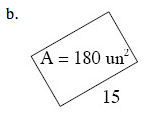Try not to be confused by the orientation of the rectangle. Solve as in part (a).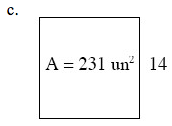See the example below.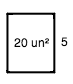$20=5\cdot \text{length}$

$\frac{ 20 }{ 5} = \frac{ 5 \cdot \text{length} }{5 }$

$\text{length} = \frac{ 20 }{ 5} = 4$